×#### Thank you for registering.

One of our academic counsellors will contact you within 1 working day.

Click to Chat

1800-1023-196

+91-120-4616500

CART 0

• 0

MY CART (5)

Use Coupon: CART20 and get 20% off on all online Study Material

ITEM
DETAILS
MRP
DISCOUNT
FINAL PRICE
Total Price: Rs.

There are no items in this cart.
Continue ShoppingTrigonometric Integrals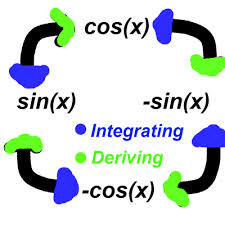Trigonometric integrals, at times can be quite challenging. There are some standard ways of dealing with trigonometric functions which help in reaching at the solutions easily and quickly. We discuss here some of the well-known standard ways of integrating trigonometric functions:

1. Integrals of the form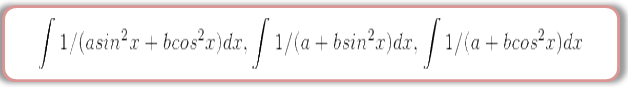The following line of action should be adopted:

(a) Both the numerator and denominator should be divided by cos2x.

(b) sec2x, wherever formed in denominator should be replaced by 1 + tan2x.

(c) Now, substitute tan x = t and so sec2x dx becomes dt.

(d) The given integral will reduce to the form

This integral can be easily solved using the methods discussed in previous sections.

Watch-this-Video-for-more-reference

2. Integrals of the form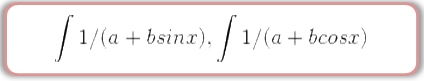In such cases, following substitutions prove fruitful:

(a) Substitute sin x by [2 tan x/2 /(1 + tan2x/2)] and cos x by (1 - tan2x/2)/(1 + tan2x/2)

(b) (1 + tan2x/2) in the numerator should be replaced by sec2x/2.

(c) Again, as in the previous case, put tan x/2 = l,so that sec2x/2 dx becomes 2dl.

(d) This again reduces the integral into the form discussed above i.e. which can be easily solved by using the methods discussed in the previous sections.

3. Integrals of the form

In such cases, students are advised to proceed as follows:

(a) Write (a sin x + b cos x) = A(c cos x – d sin x) + B(c sin x + d cos x) i.e.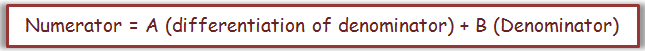(b) Now, by equating the coefficients of sin x and cos x on both the sides we can obtain the values of the two constants A and B.

(c) Now, the integrand in the numerator should be replaced by A(c cos x – d sin x) + B(c sin x + d cos x) which yields

∫ (a sin x + b cos x)/ (c sin x + d cos x) dx = A ∫ (c cos x – d sinx)/ (c sin x + d cos x) dx + B ∫ (c sin x + d cos x)/ (c sin x + d cos x) dx

= A log |c sin x + d cos x| + Bx + C

4. Integrals of the form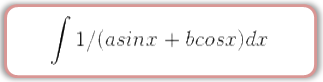In such integrals, we can use the following substitution:

(a) a = r cos θ, b = r sin θ so that r = √a2 + b2, θ = tan -1(b/a)

(b) Hence, the expression becomes a sin x + b cos x = r cos θ sin x + r sin θ cos x = r sin (x + θ)

Hence, the given integral becomes

∫1/(a sin x + b cos x) dx = 1/r ∫ 1/ sin (x + θ) dx = 1/r ∫ cosec (x + θ) dx

= 1/r log | tan (x/2 + θ/2) | + C

= 1/√a2 + b2 log | tan (x/2 + ½ tan-1b/a) | + C

5. Integrals of the formThe algorithm for this case is listed below:

(a) Write numerator as Numerator = A (differentiation of denominator) + B (denominator) + C

and so in this case we have,

a sin x + b cos x + c = A (p cos x – q sin x) + B(p sin x + q cos x + r) + C

(b) Now, try to obtain the values of constants by equating the coefficients of sin x, cos x and constants on both the sides.

(c) Replace the numerator in the integrand by A (p cos x – q sin x) + B(p sin x + q cos x + r) + C which yields

∫ (a sin x + b cos x + c)/(p sin x + q cos x + r) dx = A ∫ (p cos x – q sin x)/(p sin x + q cos x + r) dx + B ∫ (p sin x + q cos x + r)/(p sin x + q cos x + r) + C ∫ 1/(p sin x + q cos x + r) . dx

= A log | p sin x + q cos x + r| + Bx + C ∫ 1/ (p sin x + q cos x + r) dx

(d) The integral thus obtained in the R.H.S. of the last step can be obtained using the methods discussed earlier.

We have further tabulated some of the important substitutions in the below table:

Some Important FactsIntegrals of the form ∫ R ( sin x, cos x) dx:

Here R is a rational function of sin x and cos x. This can be translated into integrals of a rational function by the substitution: tan(x/2) = t. This is the so called universal substitution. In this case

sin x = 2t/1+t2 ; cos x = 1–t2 / 1+t2, x = 2 tan–1t; dx = 2dt/1+t2

Sometimes, instead of the substitution tanx/2 = t, it is more advantageous to make the substitution cot x/2 = t

Universal substitution often leads to very cumbersome calculations. Indicated below are those cases where the aim can be achieved with the aid of simpler substitutions.

(a) If R (-sin x, cos x) = -R(sin x, cos x), substitute cos x = t

(b) If R (sin x, -cos x) = -R(sin x, cos x), substitute sin x = t

(c) If R (-sin x, - cos x) = R(sin x, cos x), substitute tan x = t

Integrals of the form:

(i) ∫ p cos x + q sin x + r / a cos x + b sin x + c dx             (ii) p cosx + q sinx / a cos x + b sin x dx

Rule for (i) : In this integral express numerator as l (Denominator) + m(d.c. of denominator) + n. Find l, m, n by comparing the coefficients of sinx, cosx and constant term and split the integral into sum of three integrals.

l∫ dx + m ∫d.c. of (Denominator) / denominator dx + n ∫ dx/ a cos x + b sin x + c

Rule for (ii) : Express numerator as l (denominator) + m(d.c. of denominator) and find l and m as above

Integrals of the type: ∫(sinMx cosNx)dx

M & N ∈ natural numbers.

If one of them is odd, then substitute for term of even power.

If both are odd, substitute either of the term.

If both are even, use trigonometric identities only.

We discuss certain examples illustrating the application of these substitutions:

Example 1:

Evaluate ∫ dx / sin x (2 cos2x – 1).

Solution:

If in expression 1/sinx (2cos2x – 1) we substitute - sinx for sin x, then the integrand will change its sign. Hence, we take advantage of the substitution

t = cos x; dt = - sinx dx. This gives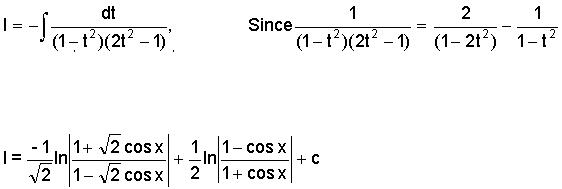____________________________________________________________________

Example 2:

Evaluate ∫ √ sin x cos x dx.

Solution:

The given question is ∫ √ sin x cos x dx.

Put sinx = t

Then, the given integral becomes

I =  ∫ t1/2 dt = 2/3 t3/2 + c = 2/3 (sin x)3/2 + c.

Example 3:

Evaluate ∫sin2 x cos4 x dx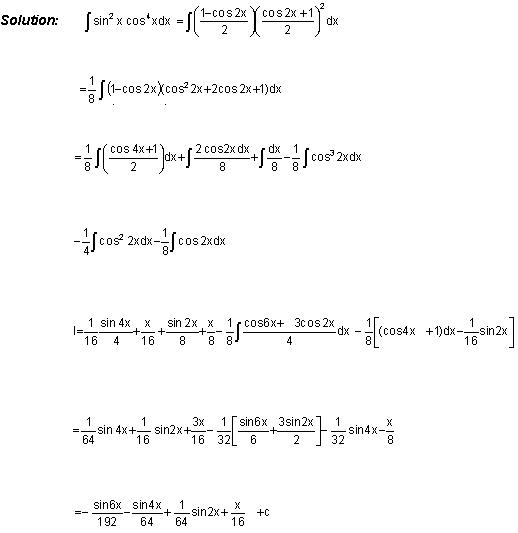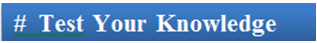Q1. In integrals of the form 1/ a sin2x + b cos2x,

(a) both the numerator and denominator should be divided by cos2x.

(b) both the numerator and denominator should be divided by sin2x.

(c) only the numerator should be divided by sin2x.

(d) only the numerator should be divided by cos2x.

Q2. While attempting to solve integrals of the form , the transformation to be used is

(a) Write denominator as a multiple of numerator.

(b) Numerator = C (differentiation of denominator) + D (denominator), C and D are constants

(c) Denominator = C (differentiation of numerator) + D (denominator), C and D are constants

(d) Numerator = C (differentiation of denominator) + D (numerator), C and D are constants

Q3. While solving rational functions involving sin x and cos x, we use the transformation tan x/2 = t. Hence, we have

(a) cos x = 2t/(1+t2), sin x = (1 - t2)/(1 + t2).

(b) sin x = 2t/(1+t2), cos x = (1 + t2)/(1 - t2).

(c) sin x = 2t/(1-t2), cos x = (1 - t2)/(1 + t2).

(d) sin x = 2t/(1+t2), cos x = (1 - t2)/(1 + t2).

Q4. In integrals of the type ∫ (a sin x + b cos x + c)/(p sin x + q cos x + r) dx,

(a) numerator = C1 (denominator) + C2 (differential of numerator) + C3

(b) numerator = C1 (denominator) + C2 (differential of denominator)

(c) denominator = C1 (numerator) + C2 (differential of denominator) + C3

(d) numerator = C1 (denominator) + C2 (differential of denominator) + C3.

Q5. While computing integrals of the type 1/(a + b cos x) or 1/(a + b sin x),

(a) substitute sin x by tan x/2.

(b) Substitute sin x by [2 cot x/2 /(1 + tan2x/2)] and cos x by (1 - tan2x/2)/(1 + tan2x/2)

(c) Substitute sin x by [2 tan x/2 /(1 + tan2x/2)] and cos x by (1 - tan2x/2)/(1 + tan2x/2)

(d) Substitute sin x by [2 tan x/2 /(1 - tan2x/2)] and cos x by (1 - tan2x/2)/(1 + tan2x/2)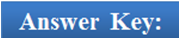Q1
Q2
Q3
Q4
Q5

(a)
(b)
(d)
(d)
(c)

Related Resources

You may wish to refer indefinite integral.

For getting an idea of the type of questions asked, refer the previous year papers.

To read more, Buy study materials of Indefinite integral comprising study notes, revision notes, video lectures, previous year solved questions etc. Also browse for more study materials on Mathematics here.### Course Features

• 731 Video Lectures
• Revision Notes
• Previous Year Papers
• Mind Map
• Study Planner
• NCERT Solutions
• Discussion Forum
• Test paper with Video Solution